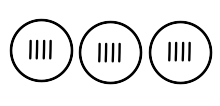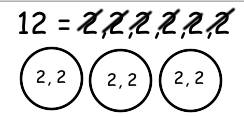# Different Ways to Divide

Instructor: Deanna Krajenke

Deanna has taught in both the general education and special education classrooms.

Division can be challenging to do. Our objective is to learn multiple strategies to help you divide whole numbers. Read on to learn about different ways to divide.

## Introduction to Division

Have you ever shared a pizza with a friend? Did you know you were actually using division to share the pizza equally so everyone got a slice? Division is used in our everyday life, so it is important to know different ways to divide so you all have the same amount of pizza!

## Vocabulary Lesson

To solve division problems, the first step is to know what each number is. There is a dividend and a divisor.

Example: 12 ÷ 3 = 4

A dividend is the total number you are dividing. In the example above, 12 is the dividend.

A divisor is the number dividing into the whole number and tells us the number of equal groups we will have. In our example, 3 is the divisor.

A whole number is a number that is not a fraction. For instance, in our example above, the number 12 is a whole number.

## Strategy 1: Making Equal Groups

1. Create the amount of circles as the divisor says. Using our same example above, you would create 3 circles.

2. Find your dividend. Using our example above, our dividend is 12. You will need this number because you will need to place one tally or dot at a time in each circle until you have a total of 12 tallies or dots (your dividend number). In other words, you would put one tally in the first circle, a second tally in the second circle, a third tally in the third circle, then back to the first circle to put your fourth tally, and so on until you have filled in a total of 12 tally marks.

3. Once all tallies have been equally divided into each group, count how many tallies are in each group. In this case, there are 4 tallies in each group, so that is your answer!## Strategy 2: Equal Groups with Place Value

This strategy is similar to Strategy 1. You will create equal groups, but instead of using tally marks, it is best to use place value.

1. Create equal groups (amount of circles of the divisor; using our example, make 3 circles).

2. Decompose your dividend into whole numbers.

3. Divide the whole numbers into the equal groups, so that all groups have the same amount of numbers in them.

4. Add up your whole numbers that are in each group. In this case, 2 + 2 = 4. So 4 is your answer!To unlock this lesson you must be a Study.com Member.

### Register to view this lesson

Are you a student or a teacher?

#### See for yourself why 30 million people use Study.com

##### Become a Study.com member and start learning now.
Back
What teachers are saying about Study.com

### Earning College Credit

Did you know… We have over 200 college courses that prepare you to earn credit by exam that is accepted by over 1,500 colleges and universities. You can test out of the first two years of college and save thousands off your degree. Anyone can earn credit-by-exam regardless of age or education level.